Students can Download Maths Chapter 3 Algebra Ex 3.2 Questions and Answers, Notes Pdf, Samacheer Kalvi 8th Maths Book Solutions Guide Pdf helps you to revise the complete Tamilnadu State Board New Syllabus and score more marks in your examinations.

## Tamilnadu Samacheer Kalvi 8th Maths Solutions Term 1 Chapter 3 Algebra Ex 3.2

Samacheerkalvi.Guru 8th Maths Question 1.
Fill in the blanks: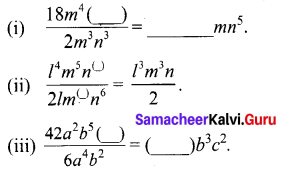Solution:
(i) $$\frac{18 m^{4}\left(n^{8}\right)}{2 m^{(3)} n^{3}}$$ = 9 mn5
(ii) $$\frac{l^{4} m^{5} n^{(7)}}{2 l m^{(3)} n^{6}}=\frac{l^{3} m^{2} n}{2}$$
(iii) $$\frac{42 a^{4} b^{5}\left(c^{2}\right)}{6(a)^{4}(b)^{2}}$$ = (7)b3c2

Samacheer Guru 8th Maths Question 2.
Say True or False:
(i) 5x3y ÷ 4x2 = 2xy
(ii) 7ab2 ÷ 14ab = 2b2
Solution:
(i) True
(ii) False

Samacheer Kalvi 8th Standard Maths Question 3.
(i) 27y3 ÷ 3y
(ii) x3y2 ÷ x2y
(iii) 45x3y2z4 ÷ (-15xyz)
(iv) (3xy)2 ÷ 9xy
Solution: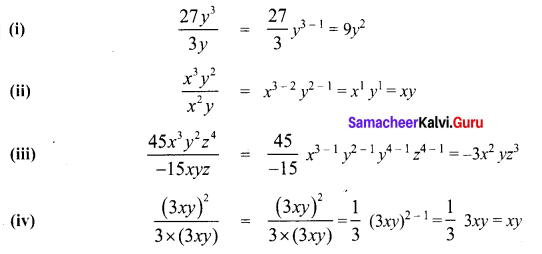Exercise 3.2 Class 8 Question 4.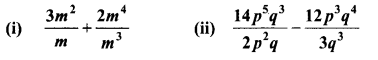Solution: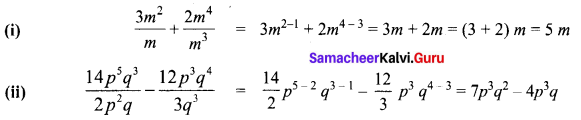Class 8 Maths Chapter 3 Exercise 3.2 Solution Question 5.
Divide
(i) 32y2 – 8yz by 2y
(ii) (4m2 n3 + 16m4 n2 – mn) by 2 mn
(iii) 10 (4x – 8y) by 5 (x – 2y)
(iv) 81 (94q2r3 + 2p3q3r2 – 5p2q2r2) by (3pqr)2
Solution: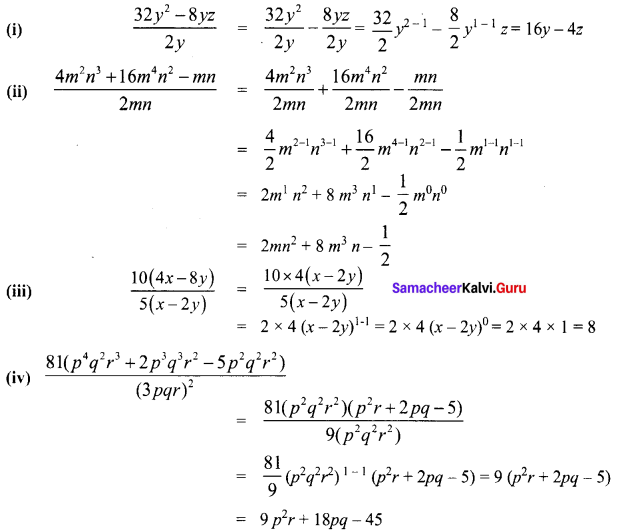Class 8 Maths Chapter 3 Notes Question 6.
Find Adirai’s percentage of marks who scored 25m3n2p out of 100m2np
Solution:
Total marks = 100 m2np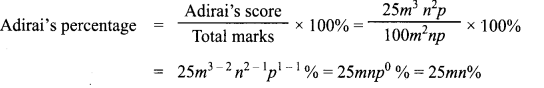Class 8 Maths Chapter 3 Exercise 3.2 Question 7.
Identify the error and correct them.
(i) 7y2 – y2 + 3y2 = 10y2
(ii) 6xy + 3xy = 9x2y2
(iii) m (4m – 3) = 4m2 – 3
(iv) (4n)2 – 2n + 3 = 4n2 – 2n + 3
(v) (x – 2) (x + 3) = x2 – 6
Solution:
(i) 7y2 – y2 + 3y2 = (7 – 1 + 3)y2 = (6 + 3)y2 = 9y2
(ii) 6xy + 3xy = (6 + 3)xy = 9xy
(iii) m (4m – 3) = m (4m) + m (-3) = 4m2 – 3m
(iv) (4n)2 – 2n + 3 = 16n2 – 2n + 3
(v) (x – 2) (x + 3) = x (x + 3) – 2 (x + 3) = x (x) + (x) × 3 + (-2) (x) + (-2) (3)
= x2 + 3x – 2x – 6 = x2 + x – 6